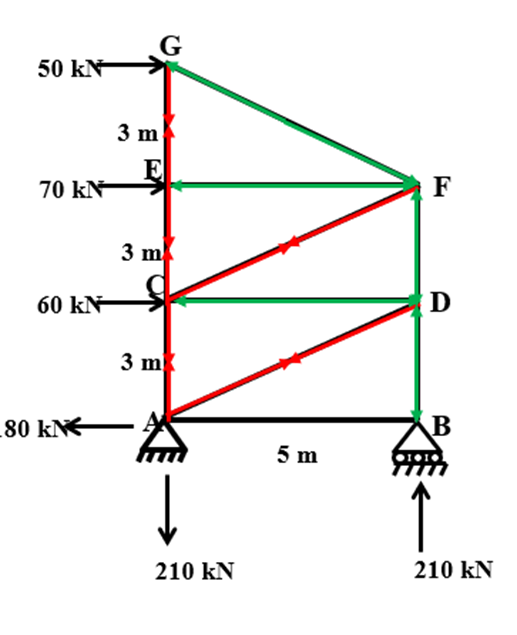### Method of Section

Method of Section

• In this method, the entire truss is divided into two parts by considering a section passing through various members.

• After determining the reactions, a section line is drawn passing through not more than three members in which forces are not known such that the frame is cut into two separate parts.

• Each part should be in equilibrium under the action of loads, reactions and forces in the members that are cut by the section line.

• Equilibrium of any one of these two parts is considered and the unknown forces in the members cut by the section line are determined.

• The system of forces acting on either part of truss constitutes a non-concurrent force system. Since there are only three independent equation of equilibrium, there should be only three unknown forces.

• The method of section is the application of non concurrent force system analysis whereas the method of joints was the application of analysis of concurrent force system.

• In a large truss in which forces in only few members are required.

• In the situation where the method of joints fails to start/proceed with analysis.

• When force in a specific member is required then this method is more suitable as compared to the method of section.

Note:
Make sure that the free body diagram of the isolated portion of the truss does not have more than three unknown forces.

Steps of Analysis

• Determine whether truss is perfect or not using the equation, m = 2j-3.

• Determine support reactions using equilibrium equations.

• Decide in which member forces are required.

• Take a section passing through a maximum of three unknown member forces which covers most of the members of step 3.

• Divide the truss into two parts using the section chosen. Consider any one part of the truss.

• Draw F.B.D of the isolated part of the truss. Assume the direction of unknown forces (Maximum 3 unknown)

• Use∑M equilibrium equation condition such that the moment of any two unknown force must be zero. Hence only one unknown force to be solved using the equilibrium equation.

• Repeat step 7 for all unknown forces of the section chosen.

Example: (Analysis of Plane Truss by Method of Section)
Determine member forces in members CE, CF and DF of the truss as shown in Figure: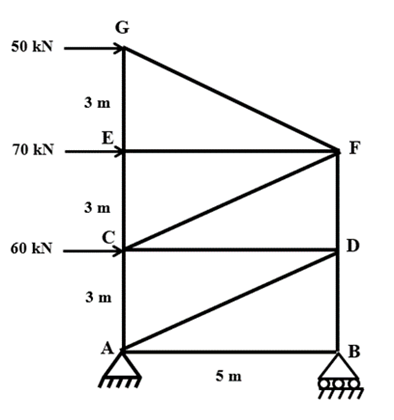Sign conventions

• Left side forces (→) are considered as +ve

• Upward Forces (↑) are considered as +ve

Step-1: To determine support reactions:
At joint A (hinge) there are two unknown reaction (HA and VA) and At Joint B there is one unknown reaction (VB). Apply equilibrium conditions: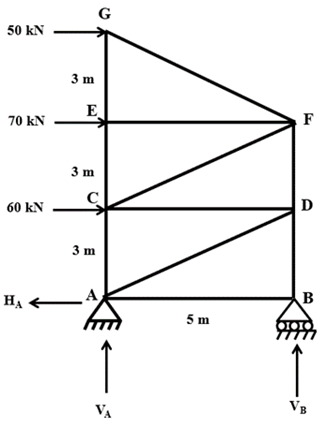Determine member forces in members CE, CF and DF of the truss as shown in Figure:
Take a section in such a way that it will be pass through all unknown members CE, CF and DF.
Cut the truss into two halves from the section.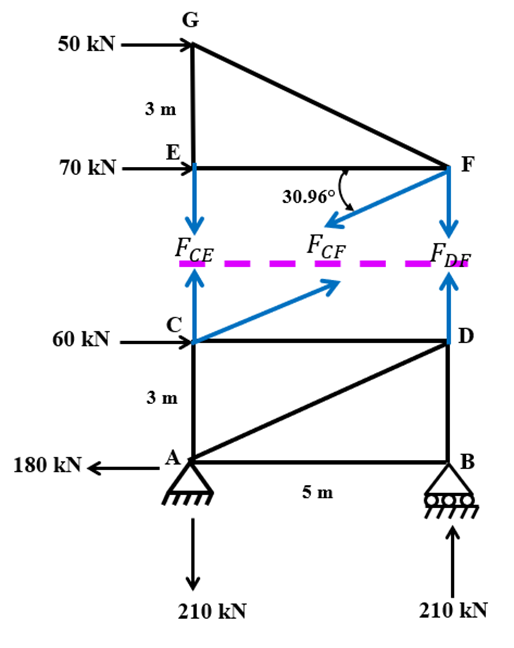Using Equilibrium conditions in any one half, determine member forces

Determine member forces in members CE, CF and DF of the truss as shown in Figure: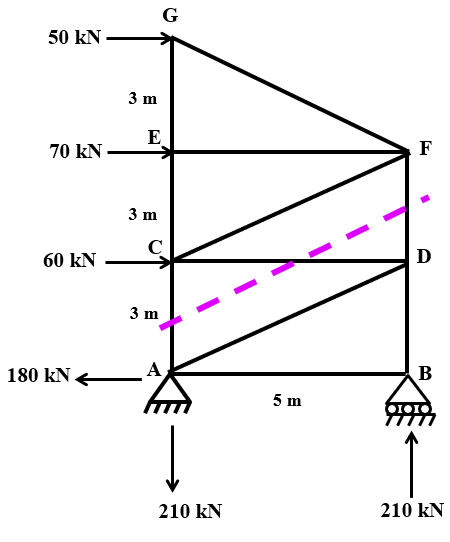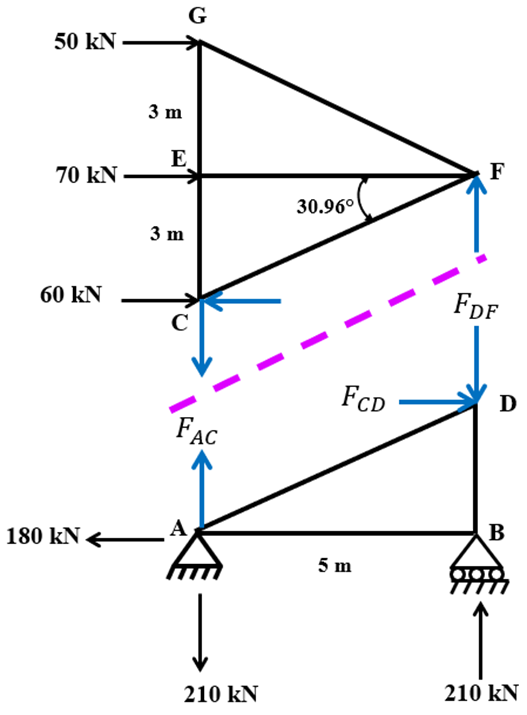Summary:

 SR.No Member Force (kN) Nature 1 AB 0 - 2 AC 102 Tension 3 AD 210 Tension 4 BD 210 Compression 5 CD 180 Compression 6 CE 30 Tension 7 CF 140 Tension 8 DF 102 Compression 9 EF 70 Compression 10 EG 30 Tension 11 FG 58.31 Compression